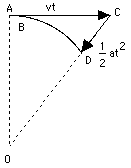# (19) Motion in a CircleIndex18. Newton's 2nd Law18a. The Third Law18b. Momentum18c. Work18d. Work against         Electric Forces19.Motion in a Circle20. Newton's Gravity21. Kepler's 3rd Law21a.Applying 3rd Law21b. Fly to Mars! (1)21c. Fly to Mars! (2)21d. Fly to Mars! (3)22.Reference Frames22a.Starlight Aberration In the absence of forces, motion in a straight line and constant velocity continues indefinitely. Motion in a circle, however, requires forces to act.     Imagine you have tied a stone to a string and are swinging it in a circle of some radius R (meters). Each rotation the stone covers a distance 2 πR meters where π = 3.14159265359. . . is the ratio between the diameter of a circle and its circumference (to memorize, count digits in "Yes, I have a super motorbike to travel about the roads foolishly").     Imagine further that the stone makes N circuits ("revolutions") per second. Since its velocity v equals the distance it moves in one second, one finds v = 2 πNR m/secIf the motion is followed for just a very brief moment, the path AB covered is so short that its curvature can be neglected, allowing one to view the motion as proceeding in a straight line, with velocity v. After a while, however, the difference between this motion and a straight-line one becomes obvious: the straight motion with velocity v would bring the particle to point C, at distance AC = vt while the actual motion brings it to some point D on the circle, whose center will be denoted by O.

In the absence of forces, motion in a straight line and constant velocity continues indefinitely. Motion in a circle, however, requires forces to act.

Imagine you have tied a stone to a string and are swinging it in a circle of some radius R (meters). Each rotation the stone covers a distance

2 πR meters

where π = 3.14159265359. . . is the ratio between the diameter of a circle and its circumference (to memorize, count digits in "Yes, I have a super motorbike to travel about the roads foolishly").

Imagine further that the stone makes N circuits ("revolutions") per second. Since its velocity v equals the distance it moves in one second, one finds

v = 2 πNR m/secIf the motion is followed for just a very brief moment, the path AB covered is so short that its curvature can be neglected, allowing one to view the motion as proceeding in a straight line, with velocity v. After a while, however, the difference between this motion and a straight-line one becomes obvious: the straight motion with velocity v would bring the particle to point C, at distance

AC = vt

while the actual motion brings it to some point D on the circle, whose center will be denoted by O.

It is useful to regard this motion as the sum of two separate motions: a straight-line motion from A to C, and then an added motion from C to D which returns the particle to the circle. As noted earlier (section on vectors), when a motion is the combination of two simpler motions, the resulting displacement can be obtained by deriving separately the displacements produced by each motion alone, then adding them together.

The added motion from C to D is the one of interest here. Its direction is always towards the center, and the distance CD covered by it--denoted here by x--can be obtained from the theorem of Pythagoras, applied to the triangle OAC (the calculation resembles the one which gave the distance to the horizon in section (8a)). In that triangle, OA = R, AC = vt, OC = R + x. Therefore

R2 + v2t2 = (R + x)2 = R2+ 2Rx + x2

Subtract R2 from both sides

v2t2 = 2Rx + x2 = x(2R + x)

If the time interval t is very short, x is much smaller than 2R and can be neglected by comparison. Then

v2t2 = 2xR

or

x = 1/2 (v2/R) t2

But by an earlier formula, in the section on acceleration, this is exactly the distance covered in time t by a motion with acceleration

a = v2/R

The above result suggests that steady motion around a circle, at least for a short time span, can be viewed as the sum of a straight-line motion with fixed velocity v, plus an accelerated motion towards the center of attraction, with the above acceleration, a.

The conclusion is correct, even though the derivation is somewhat irregular. The conventional derivation (like most of the theory of motions) requires the use of differential calculus, the study of changing quantities and of the way they change, as well as familiarity with vectors.

## Centripetal acceleration and centripetal force

The acceleration a = v2/R towards the center, needed to keep an object moving in a circle, is called its centripetal acceleration, from Latin petere, to move towards. By Newton's laws, any acceleration requires a force. If a stone (or any other object) of mass m rotates with velocity v around a central axis O, at distance R from it, a force F must constantly pull it towards the center, and

F = ma = mv2/R

This is known as the centripetal force, and by continually pulling the stone, it keeps the string stretched. If the string would break--for instance, at point A in the drawing--the stone would continue with velocity v in a straight line along AC. And no, it would not fly outwards along OA, as some believe, even though that was the direction in which the string was stretched!

Next Stop: #20 Newton's Theory of "Universal Gravitation"

Author and Curator:   Dr. David P. Stern
Mail to Dr.Stern:   stargaze("at" symbol)phy6.org .
.

Last updated 9-22-2004
Reformatted 24 March 2006